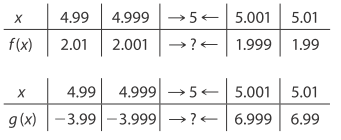# Use the given tables to evaluate the following limits, if they exist. lim x → 5 f ( x ) lim x → 5 g ( x ) lim x → 5 − g ( x )### Mathematical Applications for the ...

11th Edition
Ronald J. Harshbarger + 1 other
Publisher: Cengage Learning
ISBN: 9781305108042

#### Solutions

Chapter
Section### Mathematical Applications for the ...

11th Edition
Ronald J. Harshbarger + 1 other
Publisher: Cengage Learning
ISBN: 9781305108042
Chapter 9, Problem 8T
Textbook Problem
1 views

## Use the given tables to evaluate the following limits, if they exist. lim x → 5 f ( x ) lim x → 5 g ( x ) lim x → 5 − g ( x )This textbook solution is under construction.

### Still sussing out bartleby?

Check out a sample textbook solution.

See a sample solution

#### The Solution to Your Study Problems

Bartleby provides explanations to thousands of textbook problems written by our experts, many with advanced degrees!

Get Started